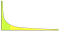# January’s most engrossing Small Data

## The Global Risks Report

As a mathematician of the old school, I don’t seek to engage Big Data. Instead, this post is about Small Data on a Big Subject. Each January since 2006 the annual Global Risks Report (GRR) has been published, as background material for the annual World Economic Forum (see Footnote 1 below). The reports are lengthy documents, freely available here analyzing risks in the sense of events that would have substantial effect on the world economy over the next few years (“medium term”). The reports provide a consensus view derived from a large panel of experts. For my purpose here, the…

# On Four Quotes By G.H. Hardy

I occasionally let slip that I am an academic great-grandchild of G.H. Hardy, whose non-technical writings about mathematics, in particular A Mathematician’s Apology are often quoted in general discussions of the nature of mathematics. Here I give my personal thoughts related to four of these well known quotations. These of course reflect my own background, with a research career in theorem-proof mathematics (albeit in the field of probability, which Hardy had a rather low opinion of) but also with interests in “real data” mathematics and in exposition. Note that Hardy writes “men”, as was the custom at the time. …

# The acid test of a generative model for data.

## How well does it predict a feature you didn’t fit?power law density (Wikipedia)

A famous 2010 paper To Explain or to Predict? by Galit Shmueli examined the essential difference between classical mathematical statistics and modern machine learning. Amazon retail uses its data on you and others to predict what you might buy, without caring why you might want to buy it — an iconic use of machine learning. In contrast a classical statistician might use (say) a multivariate Normal model merely for analytic convenience. But an implicit, and sometimes explicit, aspect of a probability model is the suggestion of causality: that observed data on smoking and lung cancer not only shows an association…

# Bayesian Updating and the “Picture Becomes Clearer” Analogy

Bayesian updating — revising an estimate when new information is available — is a key concept in data science. It seems intuitively obvious that (within an accurate probability model of a real-world phenomenon) the revised estimate will typically be better. One simple and correct mathematical formulation of “better” is that the revision can only decrease the mean squared error of the estimate.

But there is a subtlety, seldom pointed out in textbooks, which is that the actual error, while often decreasing, typically does not decrease at every “new information” step. That is, the “picture always becomes clearer” analogy is misleading.

# Doing research mathematics is like ……..

Popular writings about mathematics, and autobiographies by eminent mathematicians, provide some sense of what doing research-level mathematics is like. In this article I seek to convey the underlying implicit “flavor” of the profession, not the day-to-day explicit activity. Let’s work by analogy. That is, I will make 4 “doing research mathematics is like X” assertions, intended as a starting point for a “compare and contrast” discussion. These analogies come from my personal experience doing mathematical theory [probability, specifically] a few steps away from real world questions. …

# What is Probability For?

To start with an analogy, what is money? Textbooks and Wikipedia say that money is a medium of exchange, and a store of value, and a unit of account. But that is really the answer to a different question: “what is money for”? Asking “what is money?”, especially today when only a very small proportion of money resides in physical coins and notes rather than digital entries, is too abstruse a question for my taste.

By analogy, the question “what is probability?” is traditionally interpreted as asking for the meaning of statements such as “the probability that the home team…

# What is the significance of the Kolmogorov axioms for Mathematical Probability?

It is widely said that the Kolmogorov axioms provide the standard mathematical formalization of Probability (capitalized, to mean the discipline). This is true, but is not very informative to a non-mathematical reader, so let me explain its significance.

# Historical background.

Around 1900 the axiomatic approach to mathematics had spread well beyond its classical setting of Euclidean geometry, and the particular question of how to axiomatize Probability was highlighted as part of Hilbert’s sixth problem:

Mathematical Treatment of the Axioms of Physics. The investigations on the foundations of geometry suggest the problem: To treat in the same manner, by means of axioms, those…## David Aldous

After a research career at U.C. Berkeley, now focussed on articulating critically what mathematical probability says about the real world.